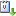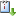Usted está aquí: Congruence testing for point sets in 4-space

# Congruence testing for point sets in 4-space

Ponente: Heuna Kim
Institución: Freie Universität Berlin
Cuándo 21/04/2015 de 17:00 a 18:00 Unidad Multidisciplinaria de Docencia e Investigación (UMDI), Aula 1. UNAM Campus Juriquilla, QuerétarovCaliCal

We give an O(n log n) time algorithm for the exact congruence testing
problem for two sets of n points in 4-space. This problem is to decide if
two point sets in the 4-dimensional Euclidean space are the same up to
rotations and translations. The problem is sensitive to numerical errors,
but since congruence testing with error tolerances is known to be NP-hard,
we restrict our concern to the exact case and use the Real Random-Access
Machine (Real-RAM) model.
For two and three dimensions, there are O(n log n) algorithms due to
Manacher (1976) and Atkinson (1987). It has been conjectured that O(n log
n) algorithms should exist for any fixed dimension. The best algorithm so
far by Brass and Knauer (2000) takes O(n^ceil(d/3) log n) time in
d-dimensional space and therefore O(n^2 log n) in 4-space.
The new algorithm makes use of properties of planes in 4-space, such as
angles, distances, and packing numbers, and also properties of rotations
in 4-space. In particular, the algorithm uses an alternative construction
of Hopf fibrations